# Numeric Selection List for Multi-Level Categoric Factors

The Half-Normal plot is preferred for selecting the model in multi-level factorial designs.

This view lists the statistical information for each estimable term.

There are five status settings: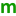- term will be considered for the model.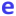- term will not be considered part of the model.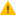- term aliased with another term and will not be considered for the model.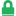- indicates that a term was manually forced into the model. It can only be removed manually.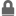- indicates that a term is required to be in the model by the program. These terms can only be removed if all other grey padlock terms are removed by fitting a mean model.

For factorial designs the default model is the mean intercept only model.

The degrees of freedom (df) is the number of pieces of information used to calculate this term’s sum of squares.

The sum of squares for a term is the amount of variation that can be attributed to the term as the factors in that term are set to their different levels.

The mean square is the sum of squares divided by the df and used to compute the F-value.

The F-value is the ratio of the term’s mean square divided by the residual’s mean square.

The Prob > For p-value is the probability that the F-value could be at least as large as it is if none of the factors had a true effect. This p-value is calculated with all estimable p-values are in the model. When insignificant terms are removed from the model, these p-values may not match what is shown on the ANOVA.

The F-value and Prob > F will be blank unless there are extra runs included in the design through replication and/or lack-of-fit runs.

A pick list of model terms is displayed. Terms with annext to them have been selected on one of the plots or have large enough effects to be automatically selected by the software. Factorial models are limited to interaction terms.

The selection can be changed by changing the order pull-down and/or double-clicking on a term to toggle anto anand vice versa. Multiple terms can be selected. Click on a starting term then hold down the shift key and click another term. All terms between the two including the ones clicked will be highlighted. Right-click on the highlighted area to set the status for the highlighted terms.

The Auto Selection opens a dialog to instruct the program to find a subset of theterms that only contains important terms. Once the automatic selection completes, click the accept button to see what choices the program made.

After the model is chosen, click on the ANOVA tab to continue the analysis and test the selected effects.